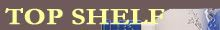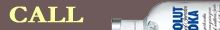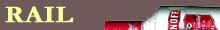Filed under:

# Award 10: The BennyBy definition, the Benny Award goes to the player most recognized for his contributions in the DCUMD blog. The award's namesake of course is Ben Olsen, the guy who everyone likes to call the talisman for DC United. Benny has meant more to this club on and off the field I think than any other player in its history. And on a day that we've all already voted our opinions on something else equally important, this is the one award with no formal voting at all. I've already got the winner in mind, and it's based on the player ratings that you've all been reading on this blog from every game throughout the entire season. Yeah, it's time for me to be a little selfish.

So with that description, and with these ratings in mind, who do you think should win the Benny Award?

Tom Soehn- C R R C R T T C C T T C R T R T R T
Zach Wells- C R C T C R C C R T R C R
Louis Crayton- T C C R R R
Jose Carvallo- R
James Thorpe- T
Bryan Namoff- R C T T T T C T T C T T R R T R T
Gonzalo Peralta- C C T T R R C R C R R R
Gonzalo Martinez- C T C C C C C R R R R C R R R T
Marc Burch- T R C R C R T T C C R R C C C R T C R C R R R T T T T
Devon McTavish- C T R C T R C C C R C C R R R R T R
Greg Janicki- T T C
Mike Zaher- T R
Pat Carroll- T R C C R
Clyde Simms- T C T R T T T C R T T R R T

Marcelo Gallardo- C T R T R R C R
Fred- T R C C C T R C R C T C T R R C R
Santino Quaranta- R T C R C C R T R T T C T T T C C R C
Joe Vide- T R T T R
Rod Dyachenko- C C R R R C R R R R C R C R
Dominic Mediate- R C R
Dan Stratford- R
Ryan Cordeiro- T C C C
Quavas Kirk- R C R T R C
Craig Thompson- C R C R C R R
Ivan Guerrero- T T C R C T T R C
Ryan Miller- C
Jaime Moreno- C T C R T T T T T T C T T T R C C
Luciano Emilio- R T R T R R C T T T T T T R T R T C R C C
Francis Doe- C T C T R T T
Thabiso Khumalo- C T R C R
Franco Niell- C R R= T= C= R
We'll dive into this more later in the week, and start announcing the winners of the Bennies next Tuesday. There's still a bit of time left to get your votes in, and some of the categories (especially the Donnety and Harkesy) are still too close to call.